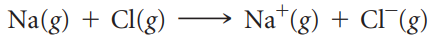×
Get Full Access to Chemistry: The Central Science - 12 Edition - Chapter 7 - Problem 109ie
Get Full Access to Chemistry: The Central Science - 12 Edition - Chapter 7 - Problem 109ie

×

# Consider the gas-phase transfer of an electron from aISBN: 9780321696724 27

## Solution for problem 109IE Chapter 7

Chemistry: The Central Science | 12th Edition

• Textbook Solutions
• 2901 Step-by-step solutions solved by professors and subject experts
• Get 24/7 help from StudySoup virtual teaching assistantsChemistry: The Central Science | 12th Edition

4 5 1 404 Reviews
24
1
Problem 109IE

Consider the gas-phase transfer of an electron from a sodium atom to a chlorine atom:(a) Write this reaction as the sum of two reactions, one that relates to an ionization energy and one that relates to an electron affinity.(b) Use the result from part (a), data in this chapter, and Hess’s law to calculate the enthalpy of the preceding reaction. Is the reaction exothermic or endothermic? (c) The reaction between sodium metal and chlorine gas is highly exothermic and produces NaCl(s), whose structure was discussed in Section 2.7. Comment on this observation relative to the calculated enthalpy for the aforementioned gas-phase reaction.

Step-by-Step Solution:
Step 1 of 3

Monday January 4, 2016 1 Important Information: ­PSet #1 due Mon (Jan 11) ­Lab Write­Up #1 due Fri (Jan 8) ­Midterm #1 January 20 Lecture 1: Thermodynamics and Kinetics Main Questions of this Course: Thermodynamics: ​ Will we ever get there Kinetics: ​ ow and when will we get there Overview ● thermodynamics gives the fundamental laws of nature → it is always right, but it may not be relevant to the question at hand ● if A → B is thermodynamically favored, then A will for B ○ may happen in 30 seconds or 30 million years ● kinetics cannot change thermodynamic outcomes ○ changing the reaction rate or pathways cannot change the thermodynamic product Reaction Rate ● reaction rate­​ tells you how much the reaction has progressed per unit time ○ can measure by looking at how the concentrations of reactants and products change over time (A → B ) ○ reaction rate = change in concentration of A / time or ○ reaction rate = change in concentration of B / time ○ the rate at which A is decreasing is the same rate at which B is increasing Clicker Question #1 Choose the correct statement for the following reaction: A + B → C a.) Reactant A must be consumed at the same rate that product C is formed b.) A is consumed at half the rate of C formation c.) A is consumed at twice th

Step 2 of 3

Step 3 of 3

##### ISBN: 9780321696724

Since the solution to 109IE from 7 chapter was answered, more than 603 students have viewed the full step-by-step answer. This textbook survival guide was created for the textbook: Chemistry: The Central Science, edition: 12. The full step-by-step solution to problem: 109IE from chapter: 7 was answered by , our top Chemistry solution expert on 04/03/17, 07:58AM. The answer to “?Consider the gas-phase transfer of an electron from a sodium atom to a chlorine atom: (a) Write this reaction as the sum of two reactions, one that relates to an ionization energy and one that relates to an electron affinity.(b) Use the result from part (a), data in this chapter, and Hess’s law to calculate the enthalpy of the preceding reaction. Is the reaction exothermic or endothermic? (c) The reaction between sodium metal and chlorine gas is highly exothermic and produces NaCl(s), whose structure was discussed in Section 2.7. Comment on this observation relative to the calculated enthalpy for the aforementioned gas-phase reaction.” is broken down into a number of easy to follow steps, and 103 words. Chemistry: The Central Science was written by and is associated to the ISBN: 9780321696724. This full solution covers the following key subjects: reaction, gas, atom, sodium, phase. This expansive textbook survival guide covers 49 chapters, and 5471 solutions.

## Discover and learn what students are asking

Calculus: Early Transcendental Functions : Antiderivatives and Indefinite Integration
?In Exercises 7–10, complete the table to find the indefinite integral. Original Integral Rewrite

Calculus: Early Transcendental Functions : Second-Order Nonhomogeneous Linear Equations
?Verifying a Solution In Exercises 1-4,verify the solution of the differential equation. Solution

Statistics: Informed Decisions Using Data : Inference about the Difference between Two Medians: Independent Samples
?In Problems 1–8, use the Mann–Whitney test to test the given hypotheses at the = 0.05 level of significance. The independent samples were obtained ra

Unlock Textbook Solution